# Venn Diagram

Go back to  'Sets'

 1 Introduction to Venn Diagram 2 What is the Venn Diagram? 3 Venn Diagram: Purpose and Benefits 4 How to Create a Venn Diagram? 5 Venn Diagram Symbols 6 Solved Examples on Venn Diagram 7 Venn Diagram Calculator 8 Important Notes on Venn Diagram 9 Practice Questions on Venn Diagram 10 Challenging Questions on Venn Diagram 11 Maths Olympiad Sample Papers 12 Frequently Asked Questions (FAQs)

## Introduction to Venn Diagram

Venn diagram was invented by John Venn.

He showed the similarities and differences between various sets visually.

## What is a Venn Diagram?

Venn diagrams in math refer to a visual representation of sets.

It shows how the items are similar and/or different.

Let's recall what we have learnt about the Number system.

Natural numbers are a subset of integers and integers are a subset of rational number and so on.

This can be represented visually for better understanding.

Here is the Venn diagram of the number system.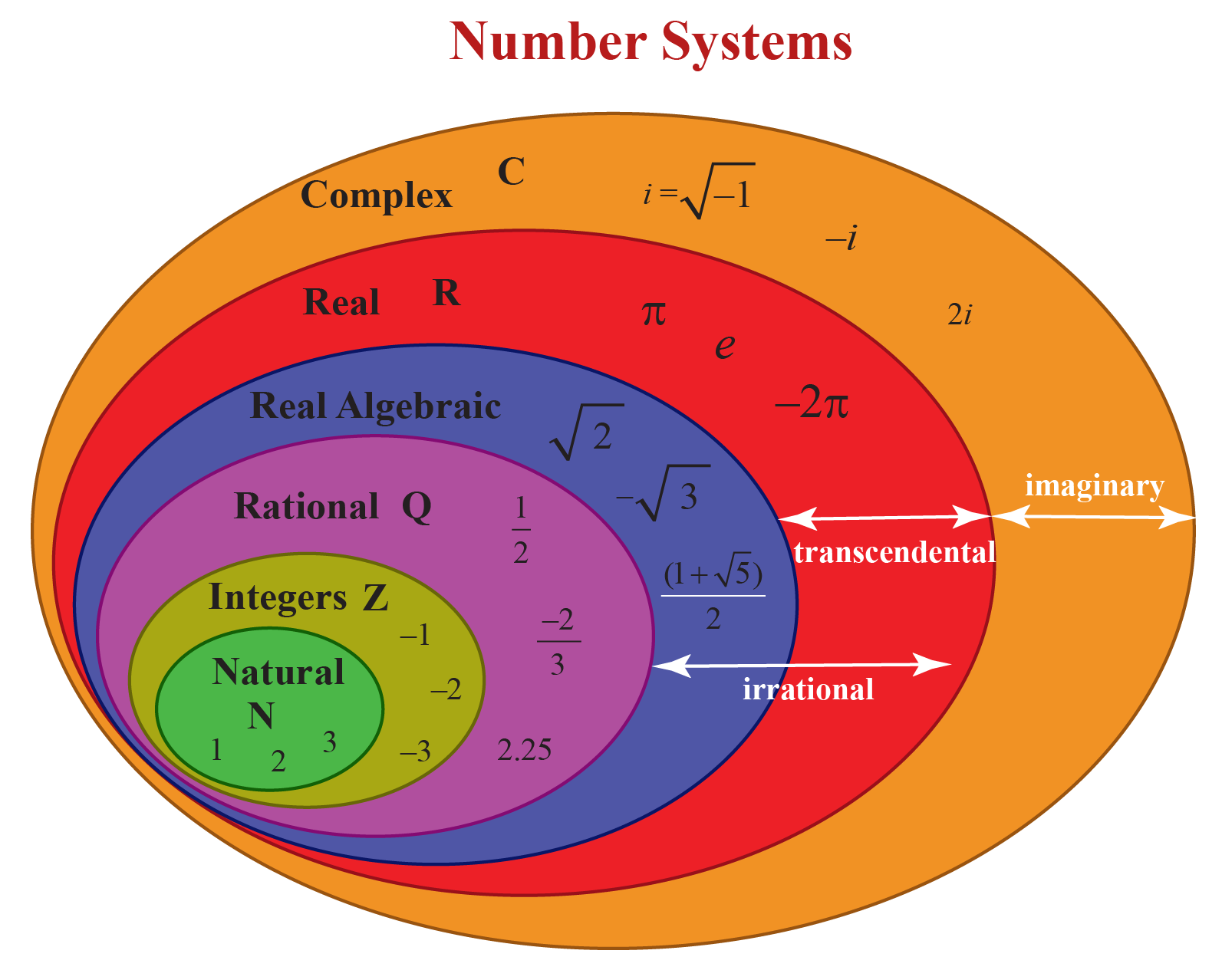We can observe that the number system is the universal set.

Natural numbers, integers, rational numbers, etc. are part of this universal set.

This Venn diagram shows how these elements are related.

## Venn Diagram: Purpose and Benefits

• They are used to visualise data clearly.
• They are used in mathematics to solve complex mathematical problems.
• They can compare two or more data easily.
• They are used in presentations and reports for decision making.
• They are used in scientific and engineering presentations.

## How to Create a Venn Diagram?

Now, let us learn how to draw a Venn diagram.

Let us create a Venn diagram for the set of animals.

We will draw a rectangle called the universe denoted by $$\text{U}$$, $$\text{V}$$ or the greek symbol $$ξ$$ which contains all the elements of the set.

Let $$\text{U}$$ be the set of animals.

It is represented as: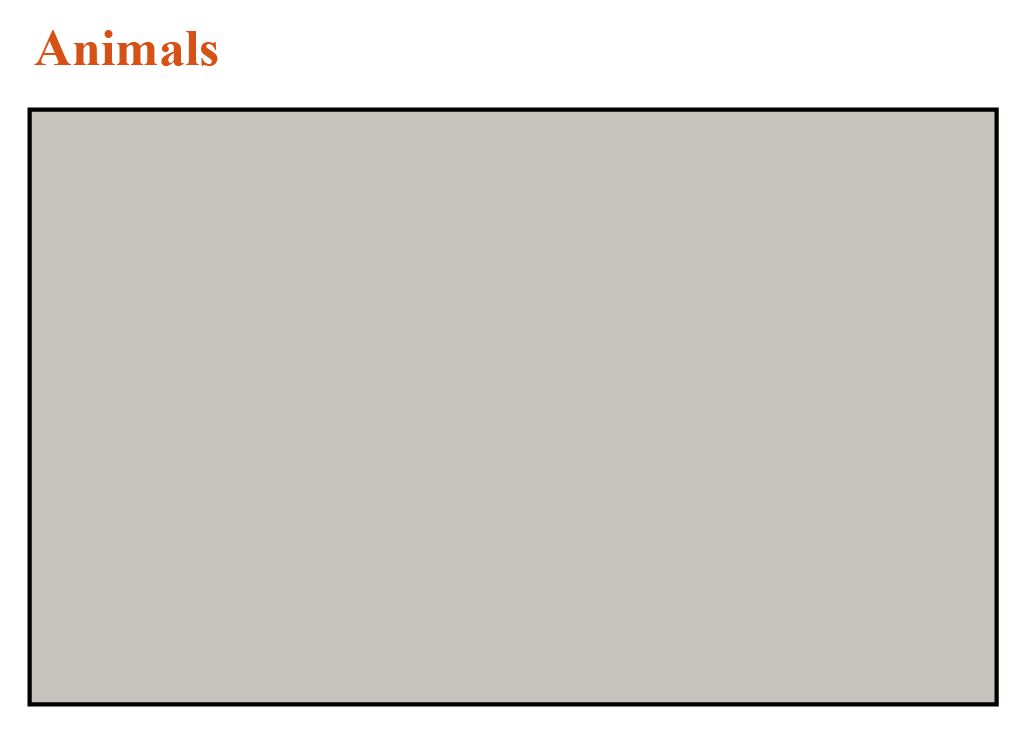Let us consider deer, rabbit, sheep, cow, horse, pig, bear, lion, tiger, cheetah, dog as the animals that are part of our set.

These animals are part of the Universal set $$\text{U}$$.

It is represented by:

$$\begin{Bmatrix} \text{Deer} & \text{Rabbit} & \text{Sheep}\\ \text{Cow} & \text{Horse} & \text{Pig}\\ \text{Bear} & \text{Lion} & \text{Tiger} \\ \text{Cheetah} & \text{Dog} \end{Bmatrix}$$

In a Venn diagram, it is represented as: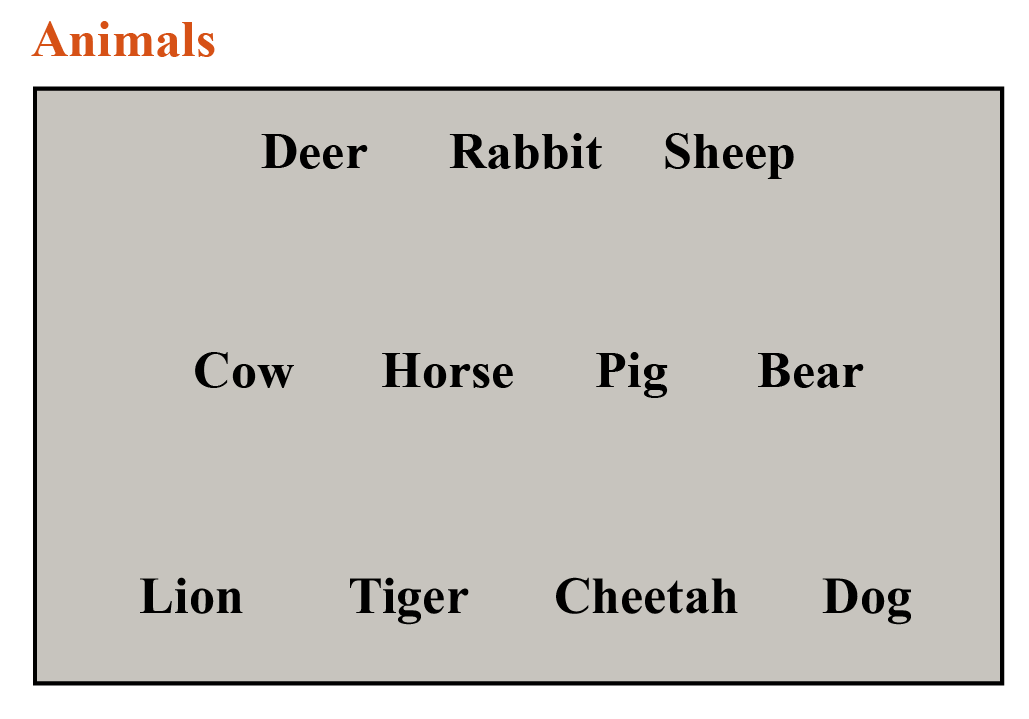From the set, we can observe that some are carnivores and some are herbivores.

Let us represent the set of herbivores by $$\text{A}$$ and carnivores by the $$\text{B}$$.

The set $$\text{A}$$ and $$\text{B}$$ are represented inside our rectangle by a circle or an oval.

Therefore,

$$\text{A} = \text{{Deer, Rabbit, Sheep, Cow, Horse}}$$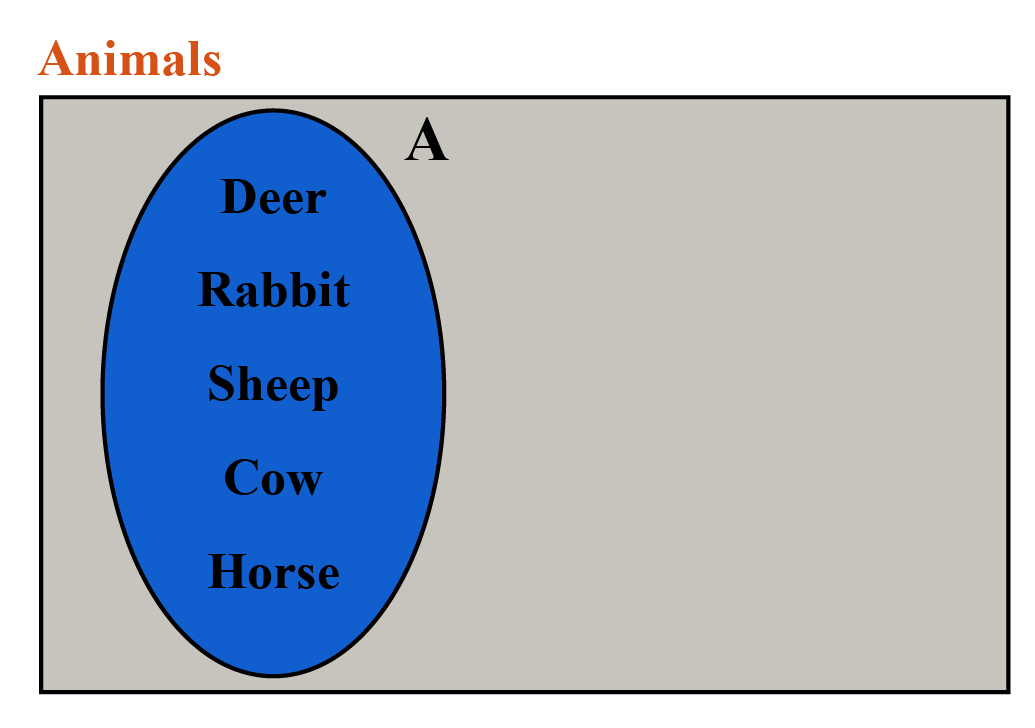$$\text{B} = \text{{Lion, Tiger, Cheetah}}$$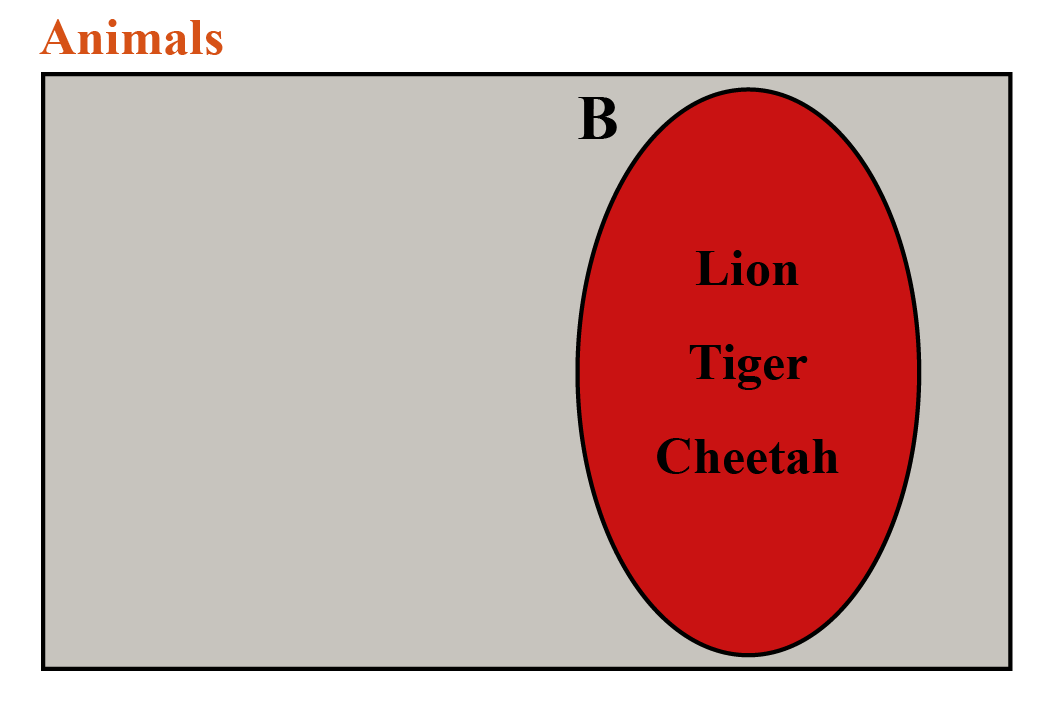Set $$\text{A}$$ and Set $$\text{B}$$ are part of the Animal set $$\text{U}$$ and are independent of each other.

We can also observe that our set $$\text{U}$$ includes $${\text {Pig, Bear, Dog}}$$.

These animals eat both plants and animals.

They are a part of set $$\text{A}$$ and set $$\text{B}$$.

We will represent these animals in our Venn diagram in this way: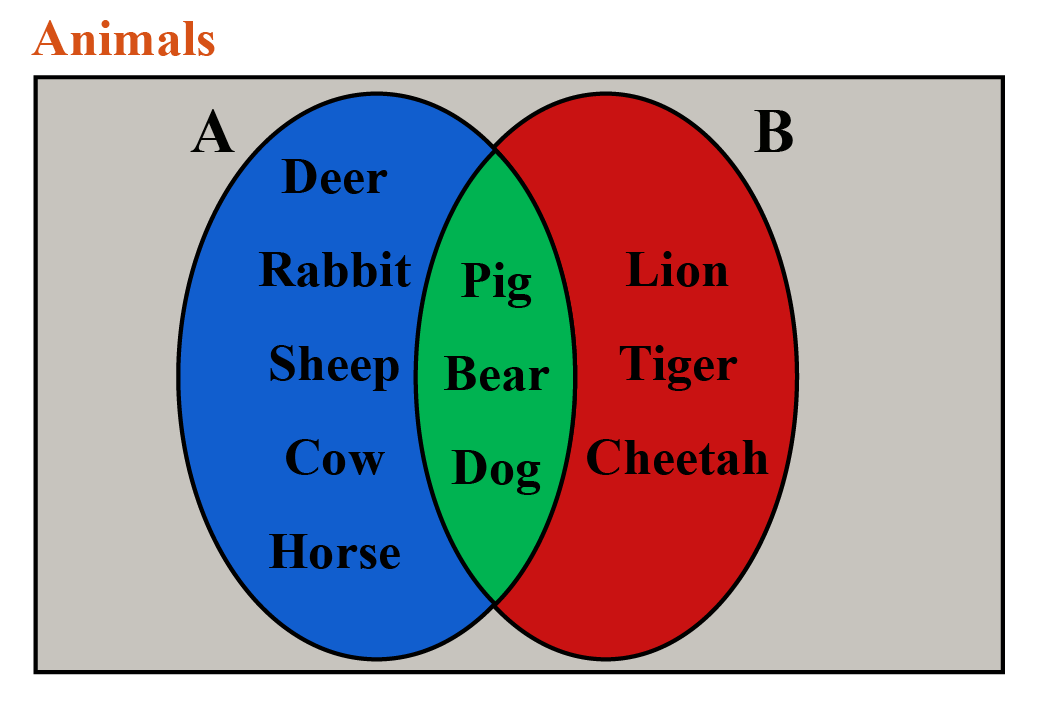The overlapping part of our Venn diagram is the Intersection.

We can also have a 3 circle Venn diagram to represent sets.

There are different set notations to help us understand Venn diagrams.

We will see more Venn diagram examples in the later sections.

## Venn Diagram Symbols

Let us consider our Universal set to have the numbers  $$\text{U}= {1,2,3,4}$$.

Subset $$\text{A}$$ includes the elements $$\text{A} = {1,2}$$ and set $$\text{B}$$ includes elements $$\text{B} = {2,3}$$.

The Venn diagram symbols for the above set are listed below in the table.

Symbol Venn Diagram Notation and  Meaning
$$\cup$$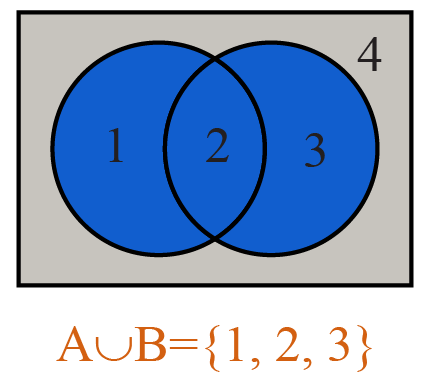$$\text{A} \cup \text{B}$$

Pronounced as $$\text{A}$$ union $$\text{B}$$

It has the elements of both $$\text{A}$$ and $$\text{B}$$

$$\cap$$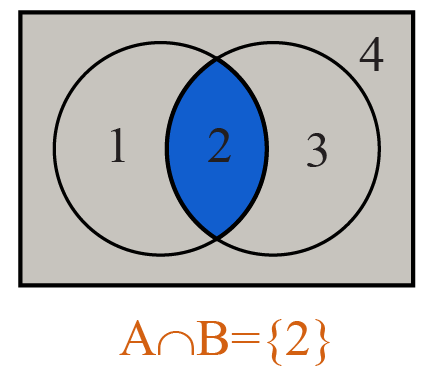$$\text{A} \cap \text{B}$$

Pronounced as $$\text{A}$$ intersection $$\text{B}$$

It has the elements common to both $$\text{A}$$ and $$\text{B}$$

$$^|$$ or $$^∁$$ or $$^\text{~}$$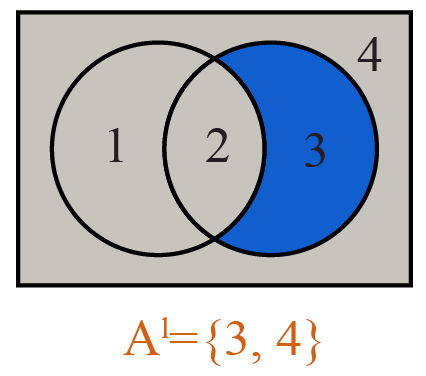$$\text{A}^|$$ or  $$\text{A}^∁$$ or  $$\text{A}^\text{~}$$

Pronounced as $$\text{A}$$ complement

All the elements that are not part of the set $$\text{A}$$

$$\text{-}$$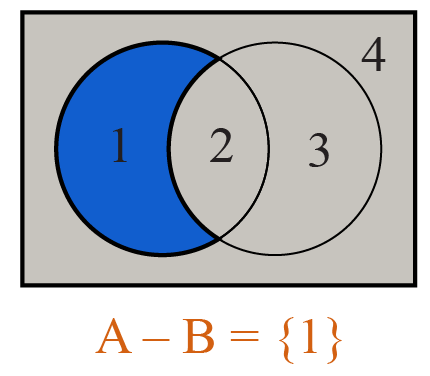$$\text{A}$$ - $$\text{B}$$

Pronounced as $$\text{A}$$ minus $$\text{B}$$

All elements that are part of only $$\text{A}$$

$$\text{⊕}$$ or  $$\Delta$$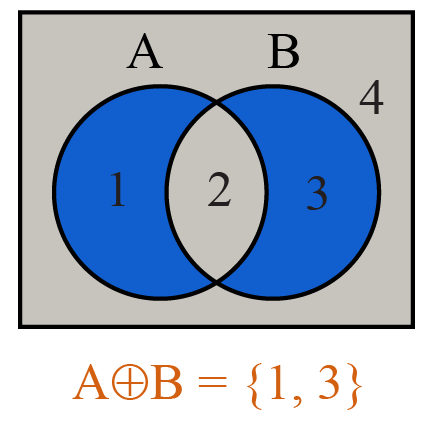$$\text{A⊕B}$$ or $$\text{A}\Delta \text{B}$$

Pronounced as symmetric difference of $$\text{A}$$ and $$\text{B}$$

All elements which belongs to exactly $$\text{A}$$ and $$\text{B}$$

## Solved Examples - Venn Diagram

Here are a few solved Venn diagram examples.

There are few questions on 3 circle Venn diagram.

Try them out on your own and verify the answers to check if you got them correct!

 Example 1

$$\text{A} = \text{{1, 3, 5, 7}}$$ and $$\text{B} = \text{{1, 2, 4, 6, 8}}$$ are two subsets of the universal set

$\text{U}= \text{{1, 2, 3, 4, 5, 6, 7, 8, 9}}$

Draw Venn diagrams to represent:

1. $$\text{A} \cup \text{B}$$
2. $$\text{A} \cap \text{B}$$
3. $$(\text{A} \cup \text{B})$$'

Solution:

$$\text{A} \cup \text{B}$$ can be represented in Venn diagram as: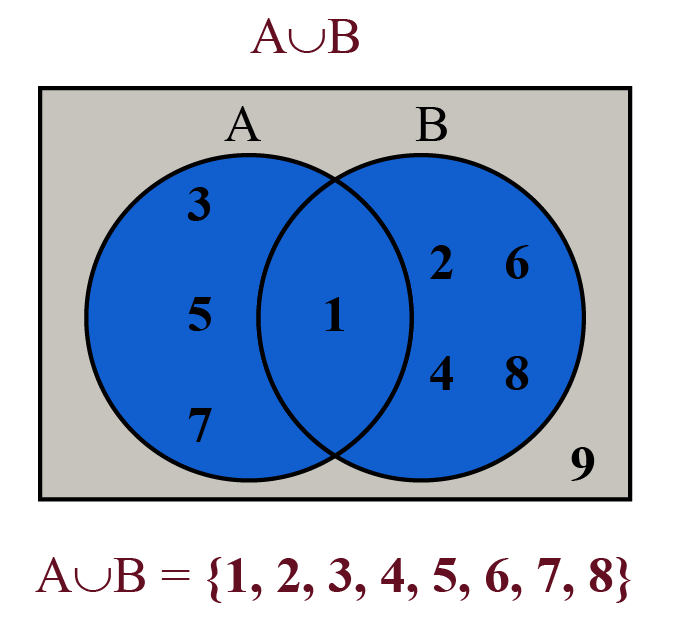\begin{align}\therefore \; \text{A} \cup \text{B} ={{1,2,3,4,5,6,7,8}} \end{align}

$$\text{A} \cap \text{B}$$ can be represented in Venn diagram as: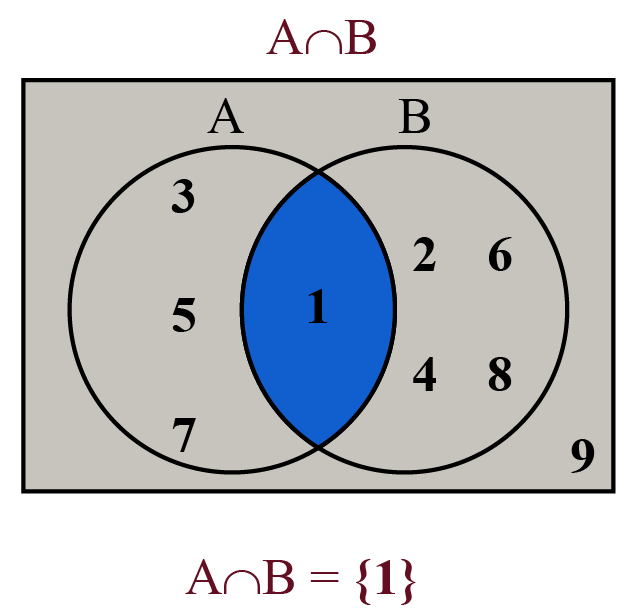\begin{align}\therefore \; \text{A} \cap \text{B} = {{1}} \end{align}

$$(\text{A} \cup \text{B})$$' can be represented in Venn diagram as: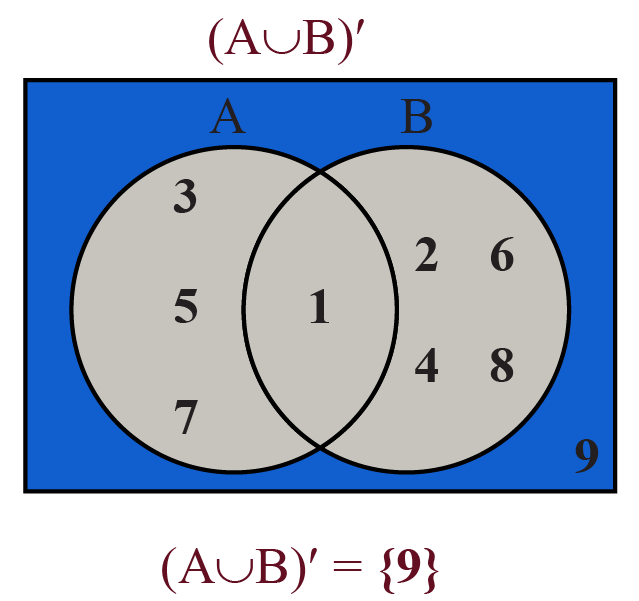\begin{align}\therefore \; (\text{A} \cup \text{B})' = {{9}} \end{align}
 Example 2

What does the shaded region in the following Venn diagram represent?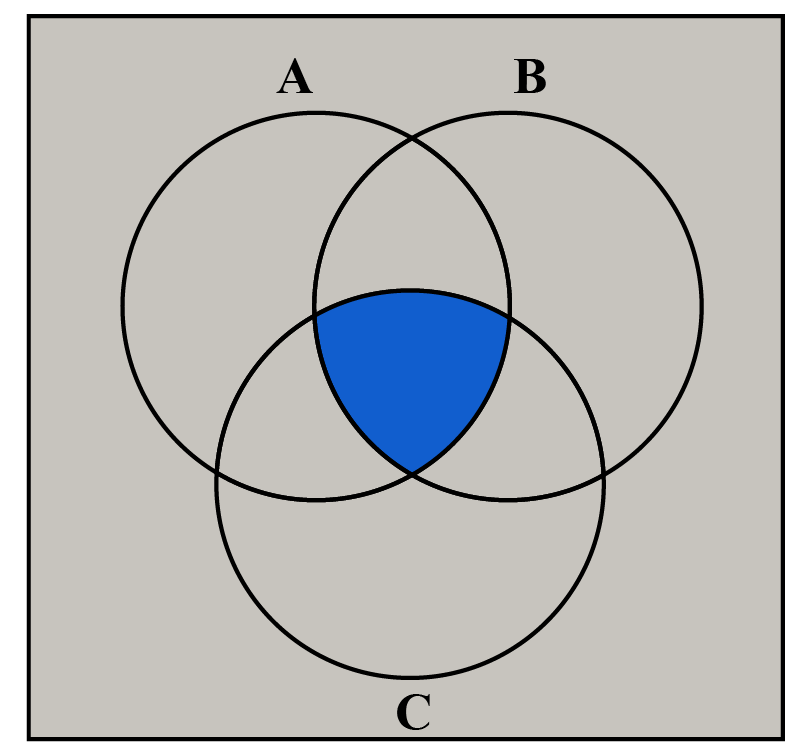Solution:

The 3 circle Venn diagrams show the common elements between the sets $$\text{A, B}$$ and $$\text{C}$$.

 Venn diagram shows $$\text{A} \cap \text{B} \cap \text{C}$$
 Example 3

In a group, $$10$$ children like pizzas and ice creams.

Out of these, $$4$$ like pizzas and $$3$$ like both pizzas and ice creams.

Represent the data in a Venn diagram and find the number of children who only like ice cream.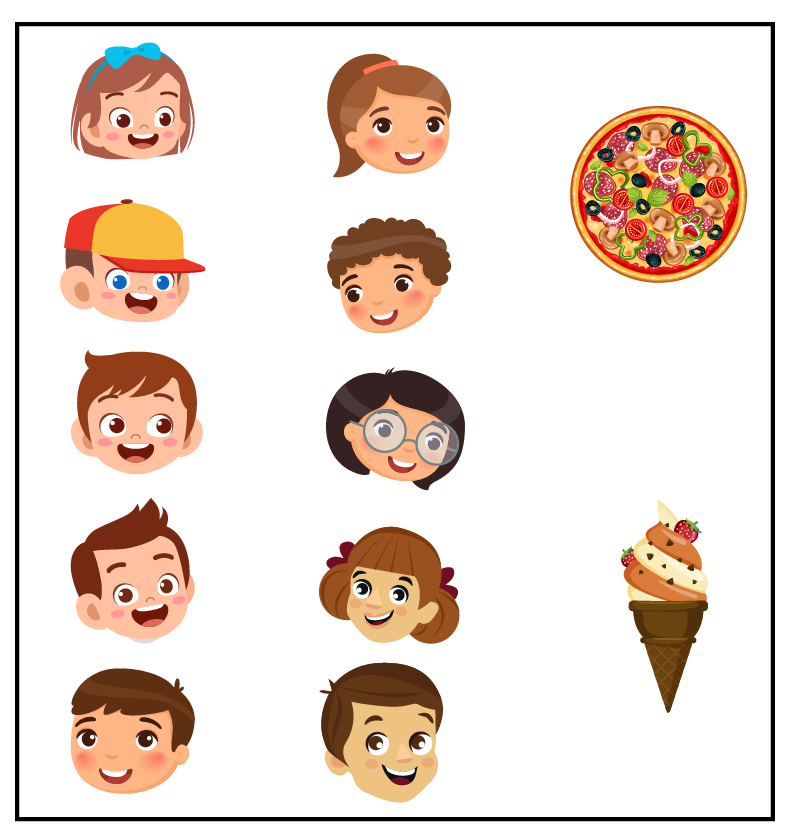Solution: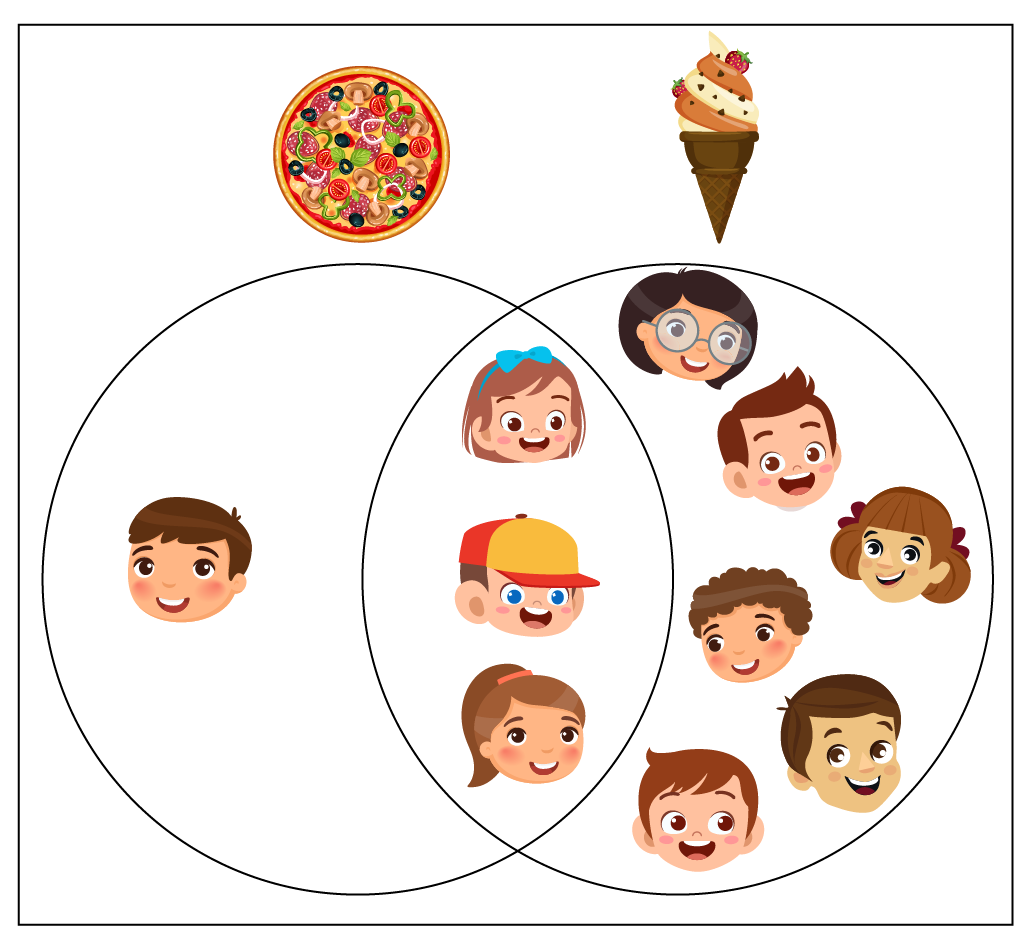From the Venn diagram, we can see that $$6$$ children only like ice cream.

 $$\therefore$$  6 children like only ice cream
 Example 4

What is the set notation for the elements $$\text{{i, f, k}}$$?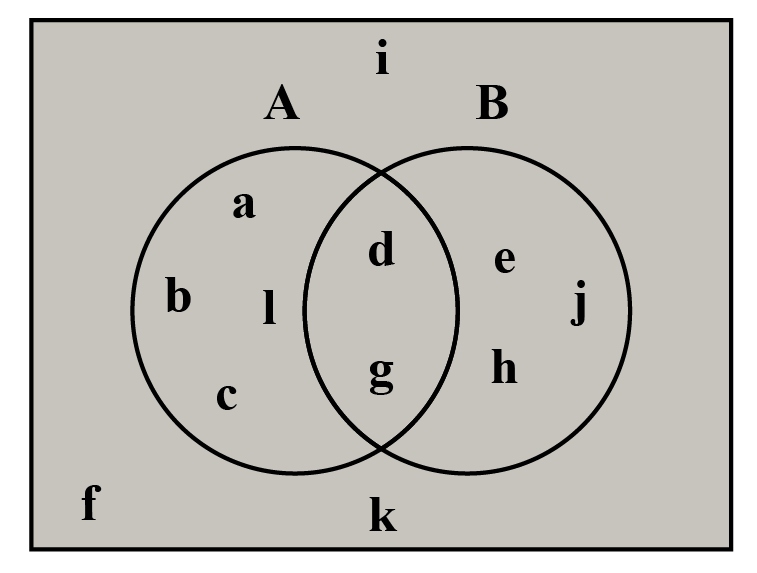Solution:

$$\text{{i,f,k}}$$ are not part of both set $$\text{A}$$ and set $$\text{B}$$.

 $$\therefore (\text{A} \cup \text{B})’$$
 Example 5

Answer the following based on the given Venn diagram.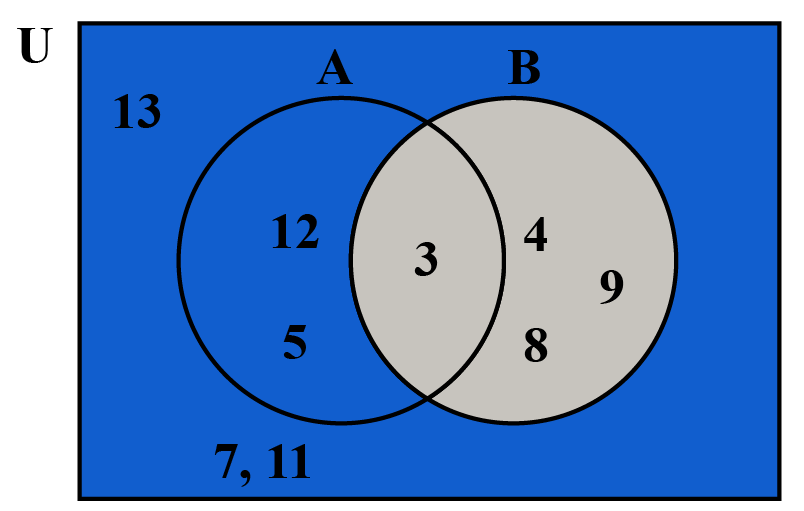List the elements of:

1. $$\text{U}$$
2. $$\text{A}^|$$
3. $$\text{B}^|$$
4. $$\text{A}^| \cup\text{B}^|$$
5. $$\text{A}^| \cap\text{B}^|$$

Solution:

1. $$\text{U = {3, 4, 5, 7, 8, 9, 11, 12, 13}}$$
2. $$\text{A}^|=\text{{4, 7, 8, 9, 11, 13}}$$
3. $$\text{B}^|=\text{{5, 7, 11, 12, 13}}$$
4. $$\text{A}^| \cup \text{B}^| = \text{{4, 5, 7, 8, 9, 11, 12, 13}}$$
5. $$\text{A}^| \cap \text{B}^| = \text{{ 7, 11, 13}}$$

## Venn Diagram Calculator

The simulation below shows the Venn diagram for the chosen set relations.

Choose the relation between the sets for which you want to see the Venn diagram.

## Practice Questions

Here are few activities for you to practice. Select/Type your answer and click the "Check Answer" button to see the result.Challenging Questions
1. $$\text{U= {a, b, c, d....j}}$$ $$\text{A = {a, b, c, d, e, f, g}}$$, $$\text{B = {b, d, f , h, i}}$$ and $$\text{C = {c, e, f, g, i}}$$.
Populate a Venn diagram and use this to find $$[(\text{A-C}) \cup\text{B}]^|$$.
2. St. Marks School held its annual talent show.
36 trophies were distributed for the music events, 12 trophies were awarded to the dance competition and 18 trophies were given to stand up comedy show.
45 students received these trophies and 4 of them got trophies in all the three events.
How many students received trophies in exactly two of these events?

IMO (International Maths Olympiad) is a competitive exam in Mathematics conducted annually for school students. It encourages children to develop their math solving skills from a competition perspective.

## 1. What is a Venn diagram and what is it used for?

A Venn diagram is a visual representation of sets.

It shows the relations between the elements of the set.

It is widely used in presentations and reports for decision making.

It is also widely used in mathematics to solve complex mathematical problems.

## 2. How do Venn diagrams work?

Venn diagrams give a visual description of the elements of the set and how they are related to each other.

It reduces the amount of text to read and understand.

It provides the user a better understanding of the relations between the elements in the set.

## 3. How do you represent the various operations using Venn diagrams?

Use the Venn diagram calculator to see the relations between the sets and the operations on them.

More Important Topics
Numbers
Algebra
Geometry
Measurement
Money
Data
Trigonometry
Calculus